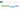# How to Join Cells In Excel with a Comma (or other delimiter)

June 29, 2015

There are times where I need to join cells in Excel and separate them with a comma or some other text. I usually need this to make a SQL query or something like this. Whatever the case may be, Excel doesn’t seem to have an easy way to join multiple cells with a delimiter like a comma. So I’ve created a very simple VBA function to help us with this task.

## The Goal

If you had cells `A1:A3` like so: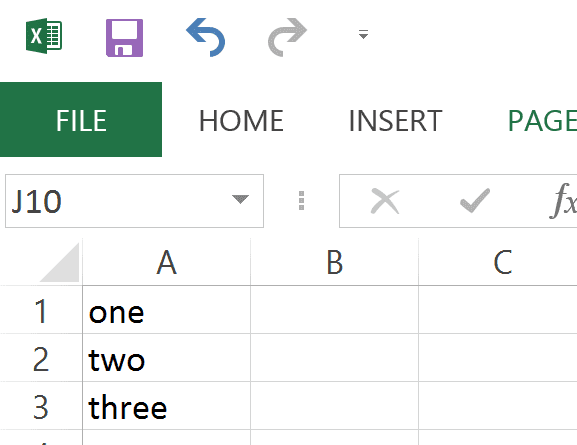And you wanted to join the text with a comma between them, one way you can do that is by using a regular formula where you concatenate the cells with ampersand (&):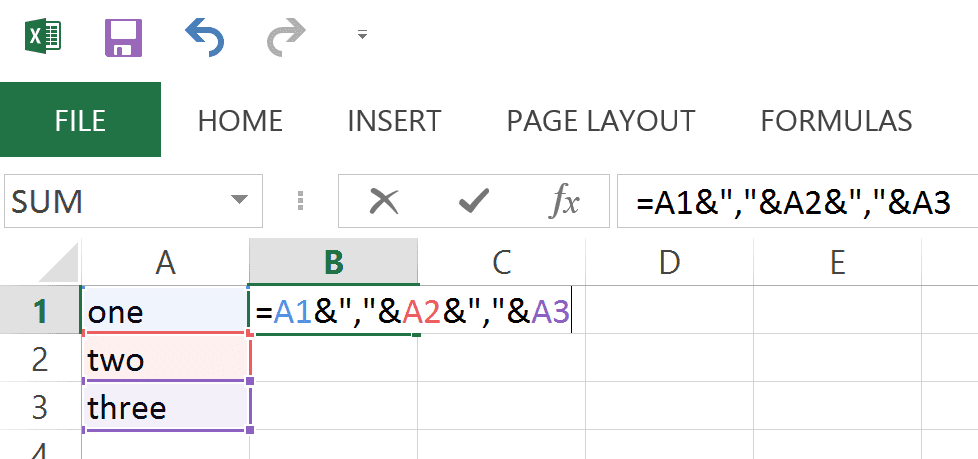However, this can become a lot of typing, especially if you have many cells you need to put together.We can make this easier by creating a custom function in VBA that we can use in our spreadsheet.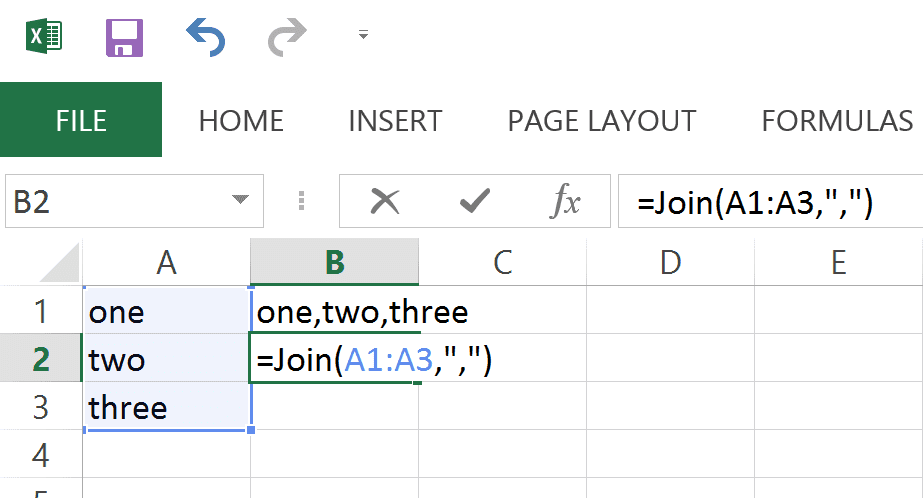[alert heading=“Note” type=“info”]There is no Join() function in Excel formulas, which is why we need to create it using VBA.[/alert]

The function will result with the same output, but with less typing: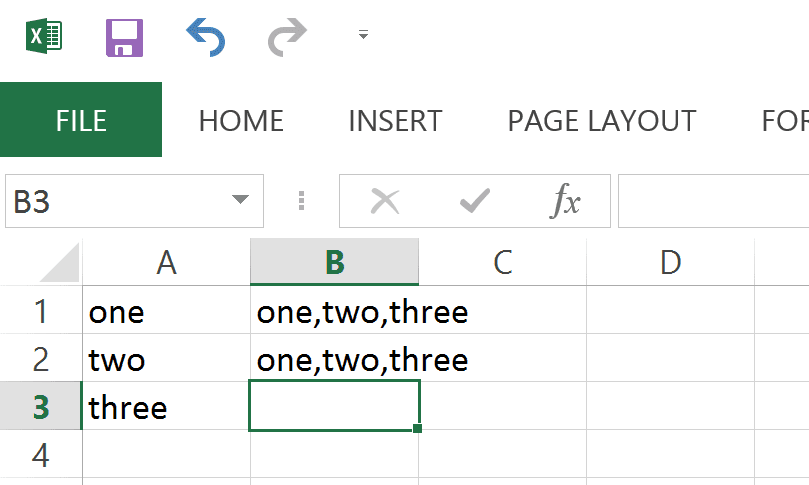Let’s create the VBA function Join() so that we can use it in our spreadsheet to make this task a lot easier.## Join Cells in Excel with a VBA Function

Let’s write a quick function you can write to help you join multiple cells with a delimiter. If you’re not very familiar with VBA, please see my Intro to VBA article.

``````Option Explicit

Public Function Join(rng As Range, delimiter As String) As String
Dim cell As Range
For Each cell In rng
Join = Join & cell.Text & delimiter
Next cell
' remove the last delimiter
Join = Left(Join, Len(Join) - Len(delimiter))
End Function``````

Let’s break this down to understand what’s going on.

``Public Function Join(rng As Range, delimiter As String) As String``

The function accepts a Cell Range as its first argument, then a string as its second argument. The function will result in a single String that will be returned to the calling Cell that uses the formula.

Here’s the next part of the function that actually builds the resulting string:

``````For Each cell In rng
Join = Join & cell.Text & delimiter
Next cell``````

Here we loop through each cell in the `rng` variable and we start building the final string by using `Join =`. Notice that we are using the name of the Function here. You can think of this as an implicit variable that Excel will use that gets returned back to the spreadsheet.

``Join = Join & cell.Text & delimiter``

Here, we build up the string cell by cell. If we had the cells:

``````A1 = one
A2 = two
A3 = three``````

And used this formula:

``=Join(A1:A3,",")``

Here would be the breakdown of how the function works.

### A1

At this point, `Join = ""`, `cell.Text = "one"`, and `delimiter = ","`.

So this line:

``Join = Join & cell.Text & delimiter``

Turns into:

``````Join = "" & "one" & ","
Join = "one,"``````

### A2

Right now, `Join = "one,"``cell.Text = "two"`, and `delimiter = ","`.

The next time we go through the line in the For Each statement, it turns into this:

``````Join = "one," & "two" & ","
Join = "one,two,"``````

### A3

And in the third time we go through the loop:

``````Join = "one,two," & "three" & ","
Join = "one,two,three,"``````

Now the loop has finished, and we have `Join = "one,two,three,"`. However, we don’t want that last comma there, so we need to get rid of it. That’s when we execute the next line:

``Join = Left(Join, Len(Join) - Len(delimiter))``

Which turns into:

``````Join = Left("one,two,three,", 14 - 1)
Join = Left("one,two,three,", 13) = "one,two,three"``````

If you’re not familiar with the `Left(string, length)` function, it’s very simple. It will take a string you give it, and only keep the amount of characters you specify. So, `Left("one,",3)` will take the string “one,” and only keep the first 3 characters (from the Left) and end up with “one”.

Also, the `Len()` function just figures out the Length a string has. So `Len("one")` will return 3, for 3 characters. (Len is short for Length).

### So why not just use Left(Join, Len(Join) - 1)?

The reason for this is if you don’t want a single character as a delimiter. Maybe instead of a comma, you want a specific piece of text between each string. For example, you could do:

``=Join(A1:A3," and ")``

Here, right before the last line we would end up with:

``Join = "one and two and three and "``

If we used:

``Left(Join, Len(Join) - 1)``

We would end up with:

``Join = "one and two and three and"``

Basically, we would only get rid of the last space at the end. This is why we should use the Length of the delimiter to remove from the end of the text. With that in mind, here’s what would happen at the end of the function:

``````Join = "one and two and three and "
Join = Left(Join, Len(Join) - Len(delimiter)
Join = Left("one and two and three and ", 26 - Len(" and "))
Join = Left("one and two and three and ", 26 - 5)
Join = Left("<strong>one and two and three</strong> and ", 21)
Join = "one and two and three"``````

[/text_output][text_output]

## Putting the New Formula to Use

To use the formula, simply type it into a cell like a regular formula:And you’re not limited to a single character for a delimiter. You can have an entire word if you want: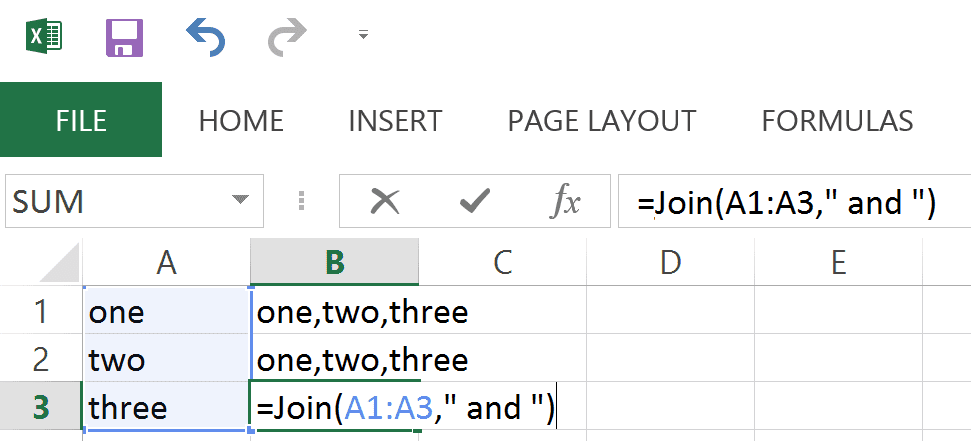Resulting in: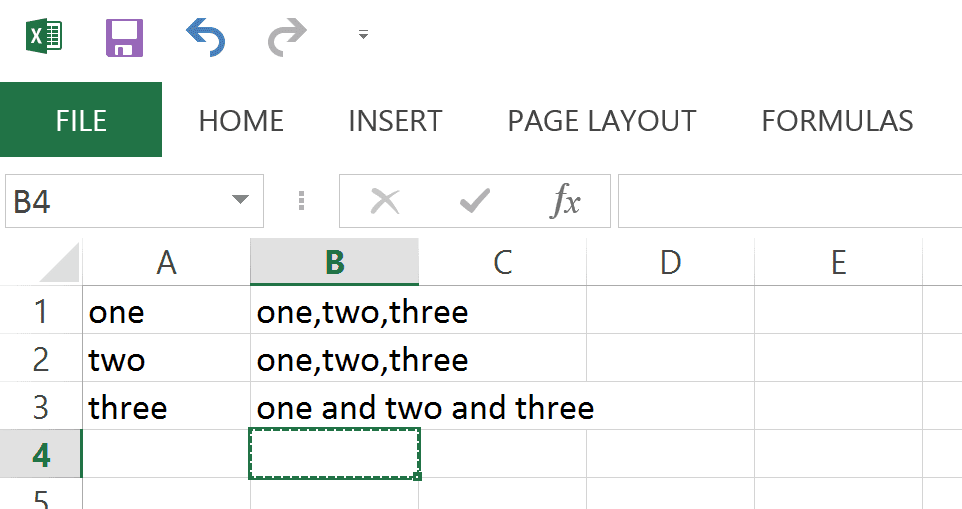I hope you found this post helpful. If you have any questions or have found a tweak to this function you’d like to share, I’d love to hear about it in the comments below!

Wow, you read the whole article! You know, people who make it this far are true learners. And clearly, you value learning. Would you like to learn more about Excel? Please consider supporting me by buying me a coffee (it takes a lot of coffee to write these articles!).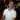Written by Joseph who loves teaching about Excel.